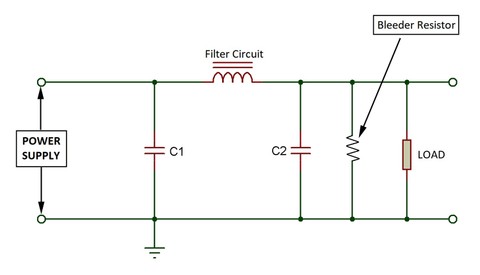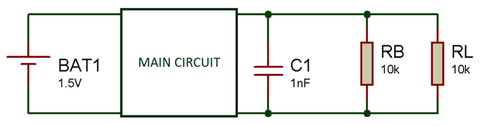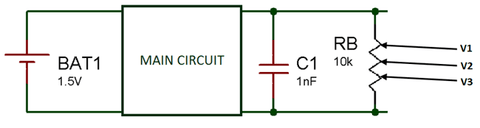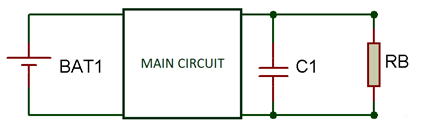# What is Bleeder Resistor and where it is used?Bleeder resistors are standard high value resistors which are used to discharge the capacitor infilter circuit. The discharging of the capacitors is really important because even if the power supply is OFF, a charged capacitor can give a shock to anybody. So it is really essential to add a bleeder resistor to avoid any mishaps. It also have other applications but the main purpose to use it is for safety purpose. In this article we will discuss how the bleeder resistor works and its applications.

### Why bleeder resistors are used?

1. Safety Purpose

Lets consider a simple circuit as shown below. Here a capacitor is attached in parallel with main circuit. Now when the power supply is ON, the capacitor will get charged to its peak value and remains charged even after the power is turned OFF, and that can be a big hazard if you are working with really high valued capacitors. This capacitor can give a high shock. So to prevent this, a resistor of a high value is connected in parallel with the capacitor, so that it may get discharged completely into the resistor.2. Voltage Regulation

Voltage regulation is the ratio of difference between full load voltage and no load voltage to the full load voltage i.e. it indicates that if a system can provide constant voltage for different loads. The formula for voltage regulation is given as:

```VR = |Vnl| - |Vfl| / |Vfl|
```

Here,

So if VR near to zero means the voltage regulation is good.

Here we connect the bleeder resistor in parallel with both capacitor and load resistor and there will also be a voltage drop across bleeder resistor. Now if the load is not connected then the no load voltage will be equal to the voltage drop across bleeder resistor. And after connecting the load, the voltage drop across the load is taken into account. So, if we connect the bleeder resistor then the difference between no load and full load voltage is quiet less which improves voltage regulation.

Lets say, If we connect the load voltage then the full voltage will 23.5V and if we remove the voltage then the voltage due to bleeder resistor is 22.4V so the voltage difference between them is 1.1V which is quiet low. Now if we do not connect the bleeder resistor then this difference will be high and hence the regulation will be low.

You can also check other methods for voltage regulation.

3. Voltage Division

This is also an important function of bleeder resistor. If you want your circuit to provide more than one or two voltages then it can be achieved by using bleeder resistor. Here the bleeder resistor is tapped at multiple points and it will act as different resistors connected in series.

In the figure below, we have tapped the bleeder resistor at three different point to get three different voltages outputs. It works on the principal of voltage divider circuit.### How to Choose the Bleeder Resistor?

One has to compromise between power consumption and the speed of bleeder resistor. A small valued resistor can provide high speed bleeding but the power consumed is higher. So it is up to the designer that how much manipulation does he wants. The resistor value must be high enough to not interfere with power supply and at the same time low enough to discharge the capacitor quickly.

The formula to calculate the value of bleeder resistor is given as:

```R = -t/C*ln(Vsafe/Vo)
```

Here

t is the time taken by the capacitor to discharge through bleeder resistor

R is the resistance of bleeder resistor

C is capacitance of the capacitor

Vsafe is the safe voltage up to which it can be discharged

Vo is initial voltage of the capacitor

Any low value can be used as for Vsafe but if we put zero there, then it will take infinite time to discharge. So, it’s a hit and trial method. Put the safe voltage and the time with which you want to discharge the capacitor and you’ll get the value of bleeder resistor.

To manipulate the power too use the below formula:

```P = Vo2/R
```

Here P is the power consumed by the bleeder resistor

Vo is the initial voltage in capacitor

R is the resistance of bleeder resistor

So after deciding how much power consumption by the bleeder resistor can be, we can find the desired value for bleeder resistor using both the above equations.

Let us consider an example.In the circuit above let us take capacitance of C1 is 4µF, the initial voltage is Vo is 1500V and the safe voltage Vsafe is 10V. If the discharge time we want is 4 seconds then the bleeder resistor value should be 997877.5 ohms or below than that. You can use a near valued resistor to this value. The power consumption will be 2.25W.

The resistor value is calculated by putting the capacitance, initial voltage, safe voltage and discharge time in the first formula. Then put the value of initial voltage and the resistor value in second formula to get the power consumption.

The resistor value can also be found in the reverse format i.e. first decide that how much power do you want it to consume and then put the power and initial voltage in second formula. So, you will get the resistor value and then use it in first formula to calculate the discharge time constant.